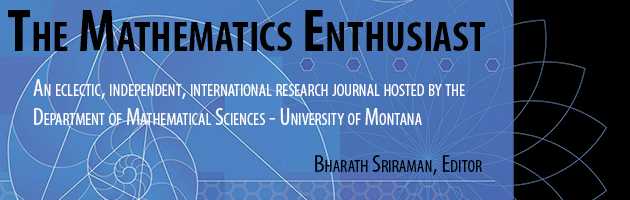•
•#### Article Title

Teaching Calculus with Infinitesimals: New Perspectives

15

3

#### Abstract

The present research corroborates K. Sullivan's initial results as stated in her epoch making study about the effectiveness of teaching elementary calculus using Robinson's non standard approach. Our research added to her results related to the teaching of the elementary integral, with similar positive results. In this essay we propose a definition of the notion of cognitive advantage mentioned by Sullivan in expressing the dramatic differences in understanding of students of non standard calculus as opposed to those of its standard counterpart. Our proposal is based on ideas of Kitcher and Kuhn and allows us to better understand the didactics of Calculus. Formally K. Sullivan's claim of an observed advantage when referring to the improved understanding of non standard calculus students (as opposed to the standard approach of Weierstrass) is a consequence to the accepted fact that mathematical truths remain the same when changes of paradigms ensue, a situation markedly different from that science. While mathematical truths remain, mathematical justifications (proofs) change dramatically and increase in complexity.

371

390

COinS